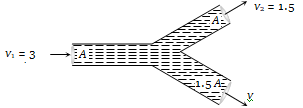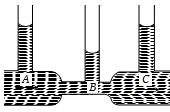Construction of submarines is based on
(a) Archimedes’ principle                (b) Bernoulli’s theorem
(c) Pascal’s law                              (d) Newton’s laws

Concept Questions :-

Archimedes principle
High Yielding Test Series + Question Bank - NEET 2020

Difficulty Level:

In which one of the following cases will the liquid flow in a pipe be most streamlined  ?

(a) Liquid of high viscosity and high density flowing through a pipe of small radius

(b) Liquid of high viscosity and low density flowing through a pipe of small radius

(c) Liquid of low viscosity and low density flowing through a pipe of large radius

(d) Liquid of low viscosity and high density flowing through a pipe of large radius

Concept Questions :-

Types of flow
High Yielding Test Series + Question Bank - NEET 2020

Difficulty Level:

Two water pipes of diameters 2 cm and 4 cm are connected with the main supply line. The velocity of flow of water in the pipe of 2 cm diameter is

(a) 4 times that in the other pipe

(b) times that in the other pipe

(c) 2 times that in the other pipe

(d) times that in the other pipe

Concept Questions :-

Equation of continuity
High Yielding Test Series + Question Bank - NEET 2020

Difficulty Level:

An incompressible liquid flows through a horizontal tube as shown in the following fig. Then the velocity v of the fluid is(a)    3.0 m/s                           (b) 1.5 m/s

(c) 1.0 m/s                             (d) 2.25 m/s

Concept Questions :-

Equation of continuity
High Yielding Test Series + Question Bank - NEET 2020

Difficulty Level:

The velocity of kerosene oil in a horizontal pipe is 5 m/s. If $\mathrm{g}=10\mathrm{m}/{\mathrm{s}}^{2}$ then the velocity head of oil will be
(a) 1.25 m                                (b) 12.5 m
(c) 0.125 m                              (d) 125 m

Concept Questions :-

Equation of continuity
High Yielding Test Series + Question Bank - NEET 2020

Difficulty Level:

In the following fig. is shown the flow of liquid through a horizontal pipe. Three tubes A, B and C are connected to the pipe. The radii of the tubes A, B and C at the junction are respectively 2 cm, 1 cm and 2 cm. It can be said that the(a) Height of the liquid in the tube A is maximum

(b) Height of the liquid in the tubes A and B is the same

(c) Height of the liquid in all the three tubes is the same

(d) Height of the liquid in the tubes A and C is the same

Concept Questions :-

Bernoulli theorem
High Yielding Test Series + Question Bank - NEET 2020

Difficulty Level:

A manometer connected to a closed tap reads . When the valve is opened, the reading of manometer falls to  , then velocity of flow of water is
(a) 100 m/s                                       (b) 10 m/s
(c) 1 m/s                                           (d) $10\sqrt{10}$ m/s

Concept Questions :-

Bernoulli theorem
High Yielding Test Series + Question Bank - NEET 2020

Difficulty Level:

A large tank filled with water to a height ‘h’ is to be emptied through a small hole at the bottom. The ratio of time taken for the level of water to fall from h to $\frac{\mathrm{h}}{2}$ and from $\frac{\mathrm{h}}{2}$ to zero is

(a) $\sqrt{2}$                                               (b) $\frac{1}{\sqrt{2}}$

(c) $\sqrt{2}-1$                                          (d) $\frac{1}{\sqrt{2}-1}$

Concept Questions :-

Bernoulli theorem
High Yielding Test Series + Question Bank - NEET 2020

Difficulty Level:

A cylinder of height 20 m is completely filled with water. The velocity of efflux of water (in m/s) through a small hole on the side wall of the cylinder near its bottom is
(a) 10                                        (b) 20
(c) 25.5                                     (d) 5

Concept Questions :-

Bernoulli theorem
High Yielding Test Series + Question Bank - NEET 2020

Difficulty Level:

There is a hole in the bottom of tank having water. If total pressure at bottom is 3 atm () then the velocity of water flowing from hole is

(a)  m/s             (b)  m/s

(c)  m/s                (d) None of these

Concept Questions :-

Bernoulli theorem